## Social Question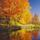# If the Earth has over 80 times the mass of the moon, why does it only have about 6 times as much gravity?

Asked by Brian1946 (27206) April 30th, 2015

According to this source, “The moon is ¼ the size of Earth, so the moon’s gravity is much less than the earth’s gravity, 83.3% (or 5/6) less to be exact.”

From what I understand about the sizes of 3-dimensional objects, the volume is the size, and the diameter of a sphere is only a single factor in determining its size.

According to my calculations, the Earth is about 50 times the size of the moon.

Also, I thought the gravitational force of an object was dependent on its mass, and not on one dimension of its size.

I.e., I’m not satisfied with the explanation I quoted.

Observing members: 0Composing members: 0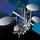The equation for gravitational attractive force is F = G * m1 * m2 / r^2. m2 is your mass. You got the mass ratio right: m1 for Earth is 80x m1 for the Moon. But when you stand on the surface, r for Moon is 1737 km but r for Earth is 6370 km. if you take the square of the distance ratio you get 0.075. Multiply by 80 => 6

RocketGuy (11604)“Great Answer” (4) Flag as…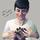So the gravitational pull you’ll feel if you’re near a planet/moon is related both to the mass of the planet (and much less significantly, your own mass – let’s assume that’s negligible here), and how far away you are from the planet’s center of mass. If measuring gravitational pull of the Earth and the moon and we assume you’re an equal distance away from each, you’re correct that their masses will be the only factor.

What I think your source was probably talking about was gravity at the surface of each, which means that radius now comes into play because you’re much closer to the center of the moon when you stand on its surface (because its radius is smaller), which means that gravity is made stronger by that. In this scenario, radius is actually more important than mass, because gravitational pull varies with the square of the radius, while it varies linearly with mass.

Mariah (25863)“Great Answer” (2) Flag as…BTW, this isn’t a homework question. ;-)

Brian1946 (27206)“Great Answer” (2) Flag as…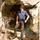You just about answered your own question. Earth has a greater gravitational force because it has a more massive iron core.

kritiper (19507)“Great Answer” (0) Flag as…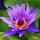BTW it might have been 55 years ago.

Tropical_Willie (26785)“Great Answer” (1) Flag as…I’m not that old. It could have been as recently as 50 years ago.

Brian1946 (27206)“Great Answer” (1) Flag as…